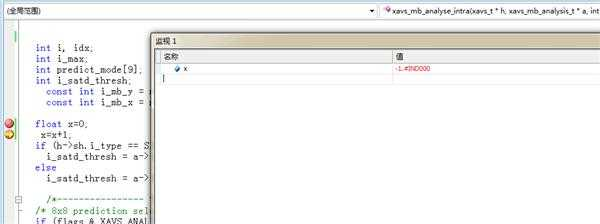﻿ 简单的关于初始化的问题 求 | Code Bye

# 简单的关于初始化的问题 求

5年前 (2016-04-25) 368次浏览
C语言的程序，原因是运行结果有问题，就拿visual一步一步监控调试，发现在刚定义了一个float型的变量时，它的值就是负无穷大……导致以后不管怎么变都是负无穷大，这可能是什么问题导致的呢？20

C++ Floating-Point Constants
Floating-point constants specify values that must have a fractional part. These values contain decimal points (.) and can contain exponents.
Syntax
floating-constant :
fractional-constant exponent-partopt floating-suffixopt
digit-sequence exponent-part floating-suffixopt
fractional-constant :
digit-sequenceopt . digit-sequence
digit-sequence .
exponent-part :
e signopt digit-sequence
E signopt digit-sequence
sign : one of
+ –
digit-sequence :
digit
digit-sequence digit
floating-suffix :one of
f l F L
Floating-point constants have a “mantissa,” which specifies the value of the number, an “exponent,” which specifies the magnitude of the number, and an optional suffix that specifies the constant’s type. The mantissa is specified as a sequence of digits followed by a period, followed by an optional sequence of digits representing the fractional part of the number. For example:
18.46
38.
The exponent, if present, specifies the magnitude of the number as a power of 10, as shown in the following example:
18.46e0      // 18.46
18.46e1      // 184.6
If an exponent is present, the trailing decimal point is unnecessary in whole numbers such as 18E0.
Floating-point constants default to type double. By using the suffixes f or l (or F or L — the suffix is not case sensitive), the constant can be specified as float or long double, respectively.
Although long double and double have the same representation, they are not the same type. For example, you can have overloaded functions like
void func( double );
and
void func( long double );

20

printf(“%lf”,x);看看
-1.#IND00 对应符号位 1 , 阶码全1 , 尾数非0 .

This page will answer the following questions.
My program just printed out 1.#IND or 1.#INF (on Windows) or nan or inf (on Linux). What happened?
How can I tell if a number is really a number and not a NaN or an infinity?
How can I find out more details at runtime about kinds of NaNs and infinities?
Do you have any sample code to show how this works?
Where can I learn more?
These questions have to do with floating point exceptions. If you get some strange non-numeric output where you”re expecting a number, you”ve either exceeded the finite limits of floating point arithmetic or you”ve asked for some result that is undefined. To keep things simple, I”ll stick to working with the double floating point type. Similar remarks hold for float types.
Debugging 1.#IND, 1.#INF, nan, and inf
If your operation would generate a larger positive number than could be stored in a double, the operation will return 1.#INF on Windows or inf on Linux. Similarly your code will return -1.#INF or -inf if the result would be a negative number too large to store in a double. Dividing a positive number by zero produces a positive infinity and dividing a negative number by zero produces a negative infinity. Example code at the end of this page will demonstrate some operations that produce infinities.
Some operations don”t make mathematical sense, such as taking the square root of a negative number. (Yes, this operation makes sense in the context of complex numbers, but a double represents a real number and so there is no double to represent the result.) The same is true for logarithms of negative numbers. Both sqrt(-1.0) and log(-1.0) would return a NaN, the generic term for a “number” that is “not a number”. Windows displays a NaN as -1.#IND (“IND” for “indeterminate”) while Linux displays nan. Other operations that would return a NaN include 0/0, 0*∞, and ∞/∞. See the sample code below for examples.
In short, if you get 1.#INF or inf, look for overflow or division by zero. If you get 1.#IND or nan, look for illegal operations. Maybe you simply have a bug. If it”s more subtle and you have something that is difficult to compute, see Avoiding Overflow, Underflow, and Loss of Precision. That article gives tricks for computing results that have intermediate steps overflow if computed directly.

CodeBye 版权所有丨如未注明 , 均为原创丨本网站采用BY-NC-SA协议进行授权 , 转载请注明简单的关于初始化的问题 求

[1034331897@qq.com]